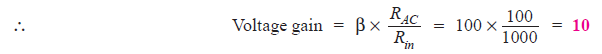# Solved Problems on Multistage Transistor Amplifiers

### Solution :

(i) Voltage gain = 20 log10 30 db = 29.54 db

(ii) Power gain = 10 log10 100 db = 20 db

### Solution :

(i) Power gain = 40 db = 4 bel

If we want to find the gain as a number, we should work from logarithm back to the original number.(ii) Power gain = 43 db = 4.3 bel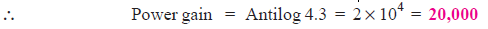### Solution :

First-stage voltage gain in db = 20 log10 100 = 20 × 2 = 40

Second-stage voltage gain in db = 20 log10 200 = 20 × 2.3 = 46

Third-stage voltage gain in db = 20 log10 400 = 20 × 2.6 = 52

∴   Total voltage gain = 40 + 46 + 52 = 138 db

### Solution :

Given : Absolute gain of each stage = 30

No. of stages = 5

(i)   Power gain of one stage in db = 10 log10 30 = 14.77

∴      Total power gain = 5 × 14.77 = 73.85 db

(ii)   Resultant power gain with negative feedback = 73.85 − 10 = 63.85 db

### Solution :

db power gain at 2 kHz :

At 2 kHz, the output power is 1.5 W and input power is 10 mW.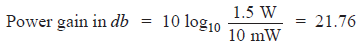db power gain at 20 Hz:

At 20Hz, the output power is 0.3 W and input power is 10 mW.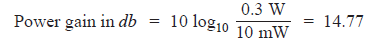Fall in gain from 2 kHz to 20 Hz = 21.76 − 14.77 = 6.99 db

### Solution :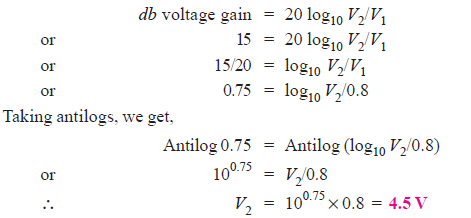### Solution :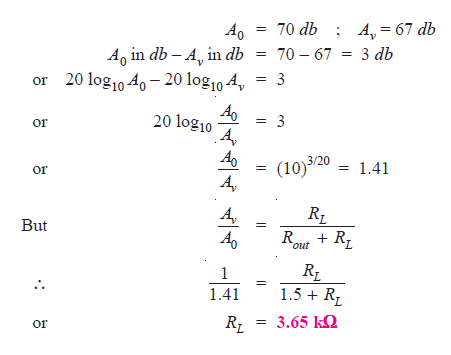### Solution :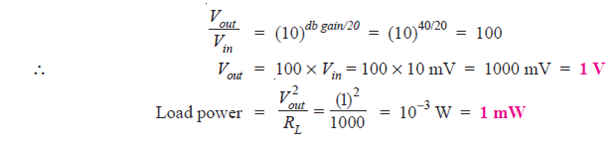### Solution :

(i)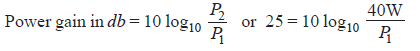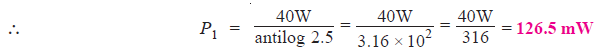(ii)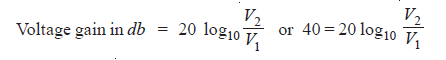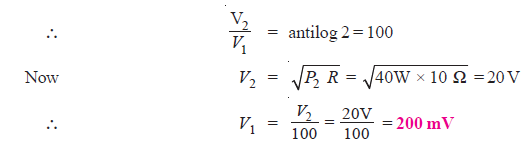### Solution :

(i) Referring to the frequency response in Fig. 1, the maximum gain is 2000.

Then 70.7% of this gain is   0.707 × 2000 = 1414

It is given that gain is 1414 at 50 Hz and 10 kHz.

As bandwidth is the range of frequency over which gain is equal or greater than 70.7% of maximum gain,

∴          Bandwidth = 50 Hz to 10 kHz

(ii) The frequency (on lower side) at which the voltage gain of the amplifier is exactly 70.7% of the maximum gain is known as lower cut-off frequency.

Referring to Fig. 1, it is clear that :

Lower cut-off frequency = 50 Hz

(iii) The frequency (on the higher side) at which the voltage gain of the amplifier is exactly 70.7% of the maximum gain is known as upper cut-off frequency.

Referring to Fig. 1, it is clear that:

Upper cut-off frequency = 10 kHz

### Solution :

The gain of second stage remains 60 because it has no loading effect of any stage.

However, the gain of first stage is less than 60 due to the loading effect of the input impedance of second stage.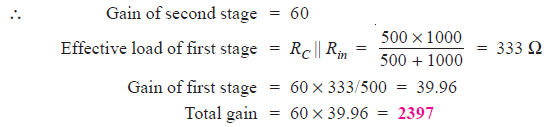### Q12. Fig. 2 shows two-stage RC coupled amplifier. If the input resistance Rin of each stage is 1kΩ, find : (i) voltage gain of first stage (ii) voltage gain of second stage (iii) total voltage gain.Fig. 2

### Solution :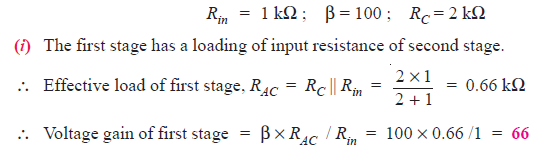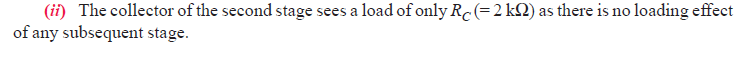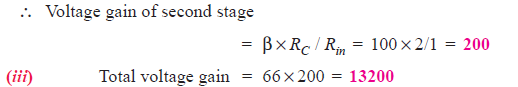### Solution :

Effective collector load, RAC = RC || RL = 10 kΩ || 100 Ω = 100 Ω